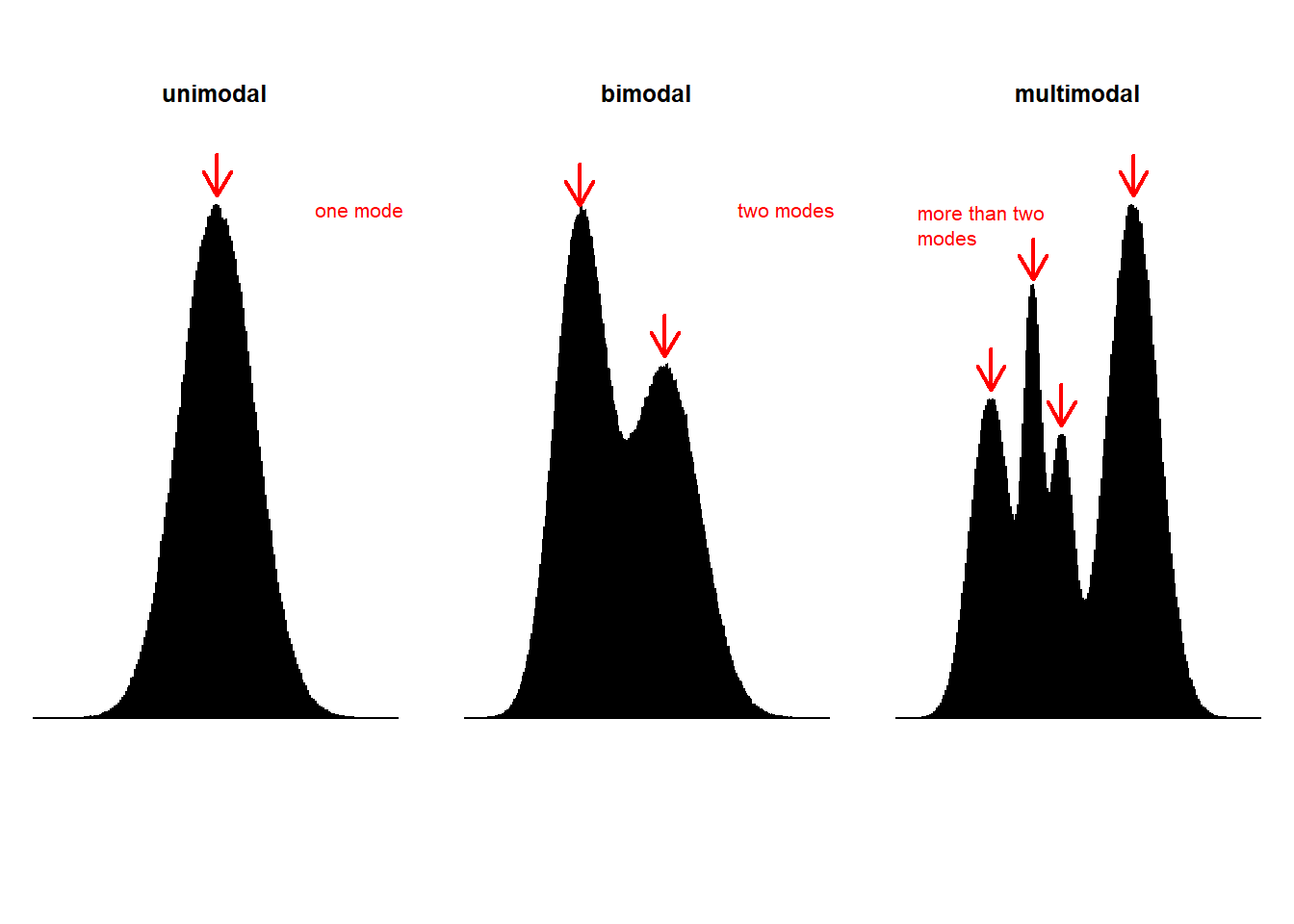In statistics, the mode represents the most common value in a data set. Therefore, the mode is the value that occurs with the highest frequency in a data set (Mann 2012). In terms of graphical frequency distribution the mode corresponds to the summit(s) of the graph.

A major shortcoming of the mode is that a data set may have none or may have more than one mode, whereas it will have only one mean and only one median. For instance, a data set with each value occurring only once has no mode. A data set with only one value occurring with the highest frequency has one mode. The data set is called unimodal in this case. A data set with two values that occur most frequently has two modes. The distribution, in this case, is said to be bimodal. If more than two values in a data set occur most frequently, then the data set contains more than two modes and it is said to be multimodal (Mann 2012).Unlike the mean and the median, the mode can be applied to quantitative (numeric) and qualitative (categorical) data. R does not have a standard in-built function to calculate the mode. So, we create a function to calculate the mode of a data set in R. This function takes a vector as input and gives the mode value as output (source code).

# Create the function
getmode <- function(v) {
uniqv <- unique(v)
uniqv[which.max(tabulate(match(v, uniqv)))]
}

Now that we have built a function, we test it on the students data set. We use the very handy apply function in order to apply the function getmode to every variable of interest. Do not forget to type help(apply) in your console if you struggle with the apply function.

students <- read.csv("https://userpage.fu-berlin.de/soga/data/raw-data/students.csv")
vars <- c("gender", "age", "religion", "nc.score", "semester", "height", "weight")
students_modes <- apply(students[vars], 2, getmode)

We may use the cbind function to print the students_modes object in a more readable column format.

cbind(students_modes)
##          students_modes
## gender   "Male"
## age      "21"
## religion "Catholic"
## nc.score "1.18"
## semester "1st"
## height   "174"
## weight   " 67.1"

Citation

The E-Learning project SOGA-R was developed at the Department of Earth Sciences by Kai Hartmann, Joachim Krois and Annette Rudolph. You can reach us via mail by soga[at]zedat.fu-berlin.de.You may use this project freely under the Creative Commons Attribution-ShareAlike 4.0 International License.

Please cite as follow: Hartmann, K., Krois, J., Rudolph, A. (2023): Statistics and Geodata Analysis using R (SOGA-R). Department of Earth Sciences, Freie Universitaet Berlin.